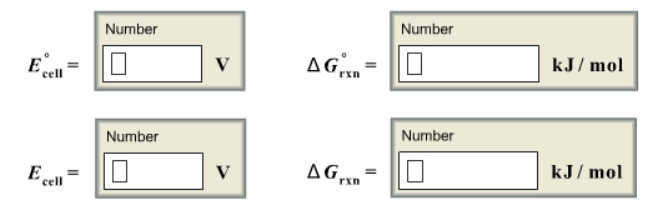# Problem: For the following electrochemical cell        Co(s) | Co2+ (aq, 0.0155 M) || Ag+ (aq, 3.50 M) | Ag(s)write the net cell equation. Phase are optional. Do not include the concentrations. Calculate the following values of 25.0 degree C using standard potentials as needed.

###### FREE Expert Solution

Co(s) | Co2+ (aq, 0.0155 M) || Ag+ (aq, 3.50 M) | Ag(s)

The Nernst Equation :

Ecell = cell potential under non-standard conditions
cell = standard cell potential
n = number of e- transferred
Q= reaction quotient = [products]/[reactants]

Step 1.

Ag+(aq) + 1 e- → Ag(s)                        E°cell = 0.80  V        → cathode
Co(s) → Co2+(aq) + 2 e-                      E°cell = -0.28 V       → anode

You can determine the anode and cathode by comparing their E° values.

• ↓ E° → oxidation → anode
• ↑ E° → reduction → cathode

Step 2. Get the overall reaction by balancing the number of electrons transferred then adding the oxidation half-reaction and reduction half-reaction.

2 Ag+(aq) + 2 e-  →  2 Ag(s)

Co(s) → Co2+(aq) + 2 e-
__________________

Co(s)2 Ag+(aq) Co2+(aq) + 2 Ag(s)

# of e- transferred = n = 2 e-

86% (318 ratings)###### Problem Details

For the following electrochemical cell

Co(s) | Co2+ (aq, 0.0155 M) || Ag+ (aq, 3.50 M) | Ag(s)

write the net cell equation. Phase are optional. Do not include the concentrations.

Calculate the following values of 25.0 degree C using standard potentials as needed.